1800-212-7858 (Toll Free)
9:00am - 8:00pm IST all days
8104911739

or

Thanks, You will receive a call shortly.
Customer Support

You are very important to us

022-62211530

Mon to Sat - 11 AM to 8 PM

# how to derive mirror equation?

Asked by Akshatha Chandrashekar 2nd October 2013, 5:33 PM

Mirror Formula (Concave Mirror)

Mirror formula is the relationship between object distance (u), image distance (v) and focal length.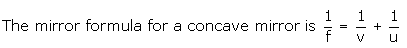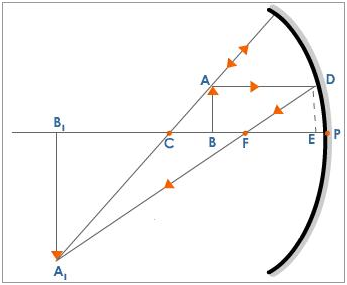## Derivation

The figure shows an object AB at a distance 'u' from the pole of a concave mirror. The image A1B1 is formed at a distance 'v' from the mirror. The position of the image is obtained by drawing a ray diagram.

Consider the D A1CB1 and D ACB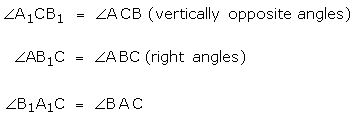[when two angles of D A1CB1 and D ACB are equal then the third angle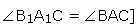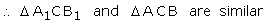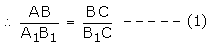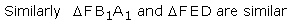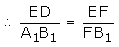But ED = AB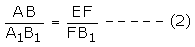From equations (1) and (2)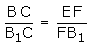If D is very close to P then EF = PF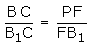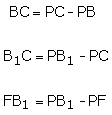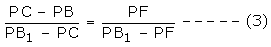But PC = R, PB = u, PB 1 = v, PF = f

By sign convention

PC = -R, PB = -u, PF = -f and PB 1 = -v

Equation (3) can be written as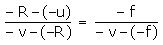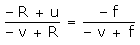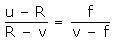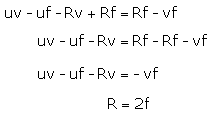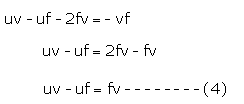Dividing equation (4) throughout by uvf we get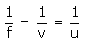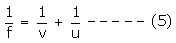Hence, equation (5) gives the mirror formula.

Answered by Expert 2nd October 2013, 7:07 PM
• 1
• 2
• 3
• 4
• 5
• 6
• 7
• 8
• 9
• 10

You have rated this answer 2/10